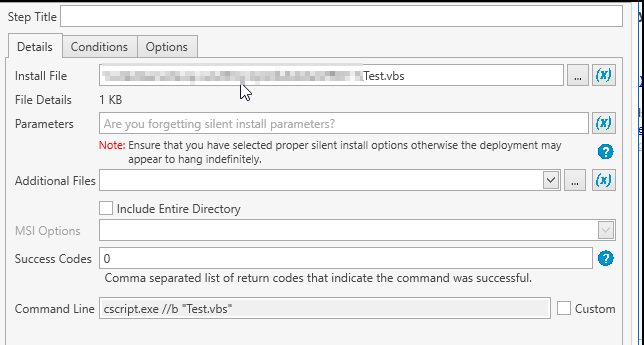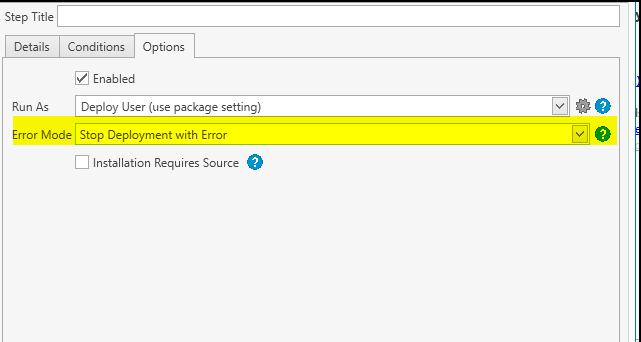# How to check if pc is a member a an AD group and if it is then don't deploy a software

Hello Im new to PDQ Deploy...i don't have PDQ Inventory so my question is

How to check if pc is a member of an AD group and if it is then don't deploy the software

Ive tried to do a PRE-Step validation by running the vbs script below but i can't get to make it work. Thanks for your help

Set WshNetwork = WScript.CreateObject("WScript.Network")
Set objFSO = CreateObject("Scripting.FileSystemObject")

If IsMember( WshNetwork.Computername,"LDAP://CN=Software - Acrobat Pro,OU=_Installation Logiciels,OU=XX,DC=XX,DC=XX,DC=XX,DC=XX") Then
'MsgBox "Member of Group"
WScript.Quit(1)
Else
'MsgBox "not Member of Group"
WScript.Quit(0)
End If
Function IsMember( sComputerName, sGroupDN )
Set group = GetObject(sGroupDN)
If IsEmpty( group.member ) Then Exit Function
sComputerName = LCase("cn=" & sComputerName)
If VarType(group.member) = vbString Then
if InStr(LCase(group.member), sComputerName ) Then
IsMember = True
End If
Exit Function
End If
For Each s In group.member
if InStr(LCase(s), sComputerName ) Then
IsMember = True
Exit Function
End If
Next
End Function1